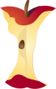Home > Grade Levels > High School Geometry >

## Cos and Sin Trigonometric Ratios#### Aligned To Common Core Standard:

Trigonometry - HSG-SRT.C.7

### Printable Worksheets And Lessons

• Cos and Sin Step-by-step Lesson - Again we go with baby steps on this one. Very fundamental in the approach that we take.

• Guided Lesson - Find the height of Jacob's building. How tall is that tree? How far is Irvin from his sticker?

• Guided Lesson Explanation - Man, I need to come up with more interesting questions. I mean; why would ever need to calculate how far you are from a sticker?

• Practice Worksheet - This sheet gets back to basics and shies away from the word problems that we just introduced.

• Matching Worksheet - This one is very deceiving. The problems are tougher than they appear.

• Find the Tangent of One Point - By about problem number 6 you should have this skill mastered.

###Answer Keys

View Answer Keys- All the answer keys in one file.

###(Click Here to Upgrade)

#### Homework Sheets

Each sheets progressively steps up in difficulty.

#### Practice Worksheets

Each sheet has two basic ratio questions and a word problem.

#### Math Skill Quizzes

The quizzes are all skill focused and not application based.

 Quiz 1 Quiz 2 Quiz 3